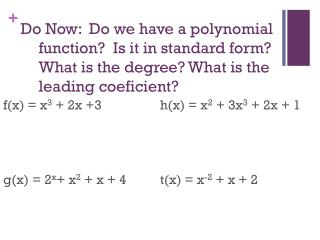DownloadDownload Presentationf(x) = x 3 + 2x +3 g(x) = 2 x + x 2 + x + 4 h(x) = x 2 + 3x 3 + 2x + 1 t(x) = x -2 + x + 2

# f(x) = x 3 + 2x +3 g(x) = 2 x + x 2 + x + 4 h(x) = x 2 + 3x 3 + 2x + 1 t(x) = x -2 + x + 2

Télécharger la présentation## f(x) = x 3 + 2x +3 g(x) = 2 x + x 2 + x + 4 h(x) = x 2 + 3x 3 + 2x + 1 t(x) = x -2 + x + 2

- - - - - - - - - - - - - - - - - - - - - - - - - - - E N D - - - - - - - - - - - - - - - - - - - - - - - - - - -
##### Presentation Transcript

1. Do Now: Do we have a polynomial function? Is it in standard form? What is the degree? What is the leading coeficient? f(x) = x3 + 2x +3 g(x) = 2x+ x2 + x + 4 h(x) = x2 + 3x3 + 2x + 1 t(x) = x-2 + x + 2

2. Chapter 2.2/2. SWBAT Evaluate and Graph Polynomial Functions Add and Subtract Polynomials

3. Solve using direct substitution! f(x) = 2x4 -5x3 -4x +8 When x = 3 Plug in 3 for all of our x values! f(3) = 2(3)4 – 5(3)3 - 4(3) + 8

4. Examples! Use direct substitution to evaluate the polynomial x4 + 2x3 +3x2 -7 ; x= -2

5. Examples! Use direct substitution to evaluate the polynomial 2 x3 + 5x2 +x +8 ; x= 3

6. Synthetic Division Steps! • Step 1: Write the coefficients of f(x) in order of descending exponents. Write the value at which f(x) is being evaluated to the left. • Step 2: Bring down the leading coefficient. Multiply the leading coefficient by the x-value. Write the product under the second coefficient. Add. • Step 3: Multiply the previous sum by the x value. Write the product under the third coefficient. Add. Repeat for all remaining coefficients. The final sum is the value of f(x).

7. Synthetic Substitution f(x) = 2x4 -5x3 -4x +8 ; x=3 3 2 -5 0 -4 8

8. Examples! Use synthetic substitution to evaluate the polynomial function for the given value of x. f(x) = 5x3 + 3x3 + x + 7 ; x= 2

9. Examples! Use synthetic substitution to evaluate the polynomial function for the given value of x. f(x) = -2x4 - x3 +4 x + 5 ; x= -1

10. 2.3 Adding and Subtracting Polynomials To add or subtract polynomials, we need to add or subtract the coefficients of like terms • For example: (2x2 +x) + (x3+3x2 –x) REORDER TO COMBINE LIKE TERMS x3 + 2x2 + 3x2 +x –x COMBINE x3 + 5x2

11. We can do this through VERTICAL or HORIZONTAL Addition Example of VERTICAL: (2x3 - 5x2 +3x -9) + (x3 +6x2 +11)

12. We can do this through VERTICAL or HORIZONTAL Addition Example of HORIZONTAL: (3x3 - 2x2 - 7x ) + (-4x2 +2x -5)

13. Lets look at HORIZONTAL SUBTRACTION (3x3 + 2x2 - x + 7) - (8x3 -x2 -5x +1)

14. Lets look at VERTICAL SUBTRACTION (5x2 - x + 3) – (4x2 + 9x -12)

15. Classwork! Guided Practice! Page 104 # 1 and # 2

16. Homework! Page 99 10-22 Even pg. 107 2-14 Even